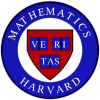Summer Tutorials 2004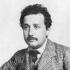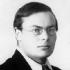Dirac Einstein Riemann Ramsey

 NOTE: This is an archived page from a previous tutorial. Information about the current tutorials can be found here.

 Welcome Message

Greetings,

I am writing to officially announce the 2004 version of our Summer Tutorial program. The idea here is to offer you some interesting mathematics if you have an ulterior motive for being in the Boston area during July and the beginning of August. In particular, the tutorials will run for six weeks meeting twice per week in the evenings (so as not to interfere with day time jobs). Three of the four will start roughly at the beginning of July and run to mid August. The fourth will start in the middle of June and run to the end of July. The precise starting dates and meeting times will be arranged for the convenience of the participants once the tutorial rosters are set.

The format will be much like that of the term time tutorials, with the tutorial leader lecturing in the first few meetings and students lecturing later on. Here, I should say that these tutorials have no official Harvard status, so you won't receive either Harvard or concentration credit for them. Moreover, enrollment in the tutorial does not qualify you for any Harvard related perks (such as a place to live). However, the Math Department will pay each student participant a stipend of \$700 and you can hand in your final paper from the tutorial for you junior 5-page paper requirement.

We are offering four tutorials this summer; the topics and leaders are as follows:

 'Riemann surfaces' lead by Deepee Khosla. 'Spinors' lead by John Francis. 'Differential geometry with a view towards physics' lead by Aaron Tievsky. 'Ramsey theory' lead by Alexander Pekker.

-- Cliff Taubes

 Tutorial: Riemann surfaces"Riemann surfaces appear across many branches of mathematics, from topology, hyperbolic geometry, and complex variables, to algebraic geometry and number theory. Indeed, a Riemann surface can be variously defined as

 a compact, complex manifold of dimension 1 a complete, non-singular algebraic curve a compact, real 2-manifold with a conformal class of metrics a compact, real 2-manifold with a metric of constant curvature a finite field extension of C(t)

In this tutorial, we will define Riemann surfaces in terms of complex manifolds. We'll see how the classical problem of computing rational integrals led Abel to consider periods on a Riemann surface. From the data of the periods, we'll constuct the Jacobian, a continuous group which generalizes the group structure on an elliptic curve and can be thought of as parametrizing the set of holomorphic line bundles. Thus armed with some technology, we'll undertake a systematic study of vector spaces of meromorphic functions on a Riemann surface. These are analytic objects which help us see more clearly the analytic (or algebraic) structure of a Riemann surface, as opposed to the topology. This will lead the way to study how a Riemann surface can be algebraically realized branched over the sphere, immersed in the complex projective plane, or embedded in complex projective space. There will be plenty of examples. Time permitting, we'll take a look how Riemann surfaces vary in families, studying various moduli and parameter spaces, each point of which represents a fixed Riemann surface.

Prerequisites: The student should be familiar with the theory of real manifolds and have some knowledge of basic complex analysis.

For further information: See the website for a syllabus and list of potential projects. Alternately, contact Deepee Khosla (deepee@math).

 Tutorial: 'Spinors'Spin lies at the heart of many exciting subjects and results in mathematics, from Dirac operators and the Atiyah-Singer index theorem to the study of classical groups and the exceptional Lie groups. Historically, in formulating the relativistic equation for the electron, Dirac needed to "squareroot" the Laplacian operator. This led to the spinors of Elie Cartan; that is, to certain representations of the Lie algebra so(n) that do not come from the special orthogonal group but rather from the Spin group, the universal cover of SO(n). Spin structures serve as a mathematical formulation of the physical notion of sub- atomic spin. Bosons have integer spin, but fermions have a "half-integer" spin and can only be modeled on a space with a spin structure, i.e., where the structure group can be lifted from the special orthogonal group to the spin group. The best tool for working with Spin is the Clifford algebra, which arises naturally as a generalization of exterior algebras when a vector space is equipped with a quadratic form. The classification of Clifford algebras underlies a lot of deep mathematics (from elliptic operators to Bott periodicity), and our goal will be to understand this classification, how to make specific interesting computations and constructions (e.g. the exceptional Lie groups), and apply these tools to topology and vector bundles. Prerequisites: Basic algebra and some familiarity with matrix groups and exterior algebras. Basic point set topology and familiarity with the fundamental group. For further information: Contact John Francis (jnfranc@fas).

 Tutorial: "Differential Geometry with a view towards physics"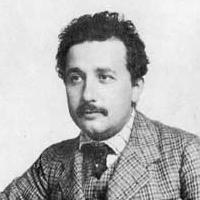In the twentieth and twenty-first centuries, theoretical physicists have made great advances that have completely changed our understanding of the universe. Many of these advances (relativity, gauge theory, string theory) rely fundamentally on the developments of modern geometry, thereby revealing an elegant geometric structure to the world that inspires physicists and mathematicians alike. In this tutorial, we will explore differential geometry and some of its amazing (and accessible) applications to theoretical physics. We will begin by discussing basic notions of differential geometry such as manifolds, tensors, connections, metrics, Riemann curvature. We will develop intuition on the one hand and computational skills on the other. This will enable us to translate freely between the mathematician's and the physicist's ways of doing differential geometry. Next, we will apply our machinery to Einstein's general theory of relativity, the truly groundbreaking theory which claims (among other things) that space-time is a smooth manifold whose curvature is determined by the presence of mass. We will develop Einstein's famous equations, emphasizing intuition and concepts over computations. We will see how black holes emerge from the theory. If time permits, we will then change gears and explore another application of differential geometry to physics, the Hamiltonian formalism of classical mechanics. After introducing the concept of a symplectic manifold, we will see how and why Hamiltonian mechanics inspired the study of symplectic geometry. Towards the end of the tutorial, the students will present lectures on topics that can range from the more mathematics oriented to the more physics oriented. Some suggestions for topics are: Vector bundles and frames, the tensorial formulation of electromagnetism, special relativity and hyperbolic geometry, more about black holes, and Lie groups (what they are and why physicists care about them.) Prerequisites: Familiarity with proofs, familiarity with basic point set topology (Math 131 is helpful, but not required) and basic knowledge of classical mechanics and special relativity as found in Physics 15a or in AP Physics C. For further information. Contact Aaron Tievsky (tievsky@math.mit.edu).

 Tutorial: "Ramsey theory"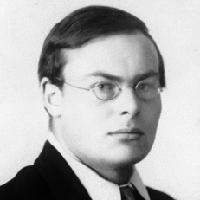The theme for this tutorial is "finding some order in enough disorder." A k-coloring of a set is simply a function that assigns to each element of the set a color numbered between 1 and k (e.g., a 2-coloring of a set is a function that assigns each element the color red or blue). Thus a coloring provides some sense of "disorder" in a set. However, we can always find some "order" provided we have enough "disorder," as the following two theorems demonstrate:

 Van der Waerden's theorem. Let k,r be positive integers. Then, there exists n>0 such that for any k-coloring of the set {1,2,3, , n}, there exists an arithmetic progress of length r in the set {1,2,3, , n} such that the elements of the arithmetic progression are all the same color. Schur's theorem. For any k-coloring of the natural numbers, the equation x+y=z has a solution such that x,y,z are all the same color.

We will develop the theory and techniques to prove these two theorems and to generalize them to much stronger results, including monochormatic solutions to systems of linear equations (Rado's Theorem) and the infinite finite-sums result (Hindman's Theorem). We will not only sharpen our counting techniques, but also apply compactness type arguments, develop new structures called filters and ultrafilters, and study what is called the *-topology of the subsets of the natural numbers. Some of these techniques also lead to new proofs of some classical results. In addition, we will study the bounds on the number n in van der Waerden's Theorem and in other results.

Here is a tentative lecture schedule: Lectures 1 and 2 will introduce monochromatic sets, Ramsey theorem, finite Ramsey theorem, canonical Ramsey theorem, Compactness arguments. Lectures 3-5 will be about Van der Waerden's theorem and Van der Waerden numbers, strengthened Van der Waerden's theorem, Ackerman's function and bounds on Van der Waerden numbers. Lectures 6-8 will be on Schur's theorem, partition-regular equations, the columns property, Rado's theorem. Lectures 9-12 will introduce filters, ultrafilters, and Hindman's theorem. There will also be lectures on infinite Ramsey theory if time permits. Note that this tutorial will run from approximately June 15 to July 31.

Prerequisites: Some knowledge of topology at the level of Math 25, 55,101, or 112.

For further information: Contact Alexander Pekker (apekker@math)

 Archive: Old Summer Tutorials since 2001

 Last update, 3/22/2004